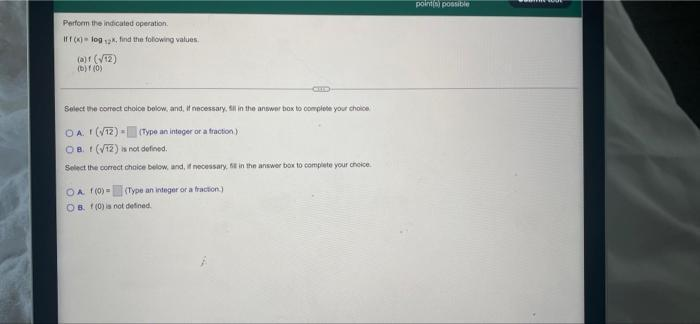Question:

# Perform the indicated operation If f (x) log 2x, find the following values. (a)f (√12) (b)f (0) CHILD Select the correct choice below, and, if necessary, fill in the answer box to complete your choicePerform the indicated operation If f (x) log 2x, find the following values. (a)f (√12) (b)f (0) CHILD Select the correct choice below, and, if necessary, fill in the answer box to complete your choice OA. (√12) = (Type an integer or a fraction) OB. 1 (√12) is not defined. Select the correct choice below, and, if necessary, 58 in the answer box to complete your choice. OA f(0) = (Type an integer or a fraction) OB. f (0) is not defined. points) possible